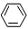Click to Chat

1800-1023-196

+91-120-4616500

CART 0

• 0

MY CART (5)

Use Coupon: CART20 and get 20% off on all online Study Material

ITEM
DETAILS
MRP
DISCOUNT
FINAL PRICE
Total Price: Rs.

There are no items in this cart.
Continue Shopping• Complete JEE Main/Advanced Course and Test Series
• OFFERED PRICE: Rs. 15,900
• View Details

```IIT-JEE-Chemistry-Mains-2003

Mains

_______________________________________________________________

Note : Question number 1 to 10 caries 2 marks each and 11 to 20 carries 4 marks each.

1.         Calculate the molarity of water if its density is 1000 kg/m3.

2.         The average velocity of gas molecules is 400 m/sec. Calculate its rms velocity at the same temperature.

3.         Write down the heterogeneous catalyst involved in the polymerization of ethylene.

4.         Which one is more soluble in diethyl ether anhydrous AICI3 or hydrous AICI3? Explain in terms of bonding.

5.         Using VSEPR theory, draw the shape of PCI5 and BrF5.

6.         A racemic mixture of (+) 2-phenyl propanoic acid on esterification with (+)
2-butanol gives two esters. Mention the stereochemistry of the two esters produced.

7.         Wavelengths of high energy transition of H-atoms is 91.2nm. Calculate the corresponding wavelength of He atoms.

8.         Match the Ka values

Ka

(a) Benzoic acid

(b) O2N--COOH

(c) CI--COOH

(d) H3CO--COOH

(e) H3C--COOH

3.3 × 10-5

6.3 × 10-5

30.6 × 10-5

6.4 × 10-5

4.2 × 10-5

9.         Write down reactions involved in the extraction of Pb. What is the oxidation number of lead in litharge?

10.       Following two aminoacids liosine and glutamine form dipeptide linkage. What are two possible dipeptides?

NH2                          NH2
|                             |
/\/\/\            +              /\/\
H2N       COOH             HOOC     COOH

11.       (a) You are given marbles of diameter 10 mm. They are to be placed such that their centres are lying in a square bound by four lines each of length 40 mm. What will be the arrangements of marbles in a plane so that maximum number of marbles can be placed inside the area? Sketch the diagram and derive expression for the number of molecules per unit area.

(b) 1 gm of charcoal adsorbs 100 ml 0.5 MCH3COOH to form a monolayer, and thereby the molarity of CH3COOH reduces to 0.49. Calculate the surface area of the charcoal adsorbed by each molecule of acetic acid. Surface area of charcoal = 3.01 × 102 m2/gm.

12.       (a) Will the pH of water be same at 4oC and 25oC? Explain.
(b) Two students use same stock solution of ZnSO4 and a solution of CuSO4. The emf of one cell is 0.03 V higher than the other. The conc. of CuSO4 in the cell with higher emf value is 0.5 M. Find out the conc. of CuSO4 in the other cell (2.203 RT/F = 0.06).

13.       Convert
F
/
(a)          COOH-→    COOH-(in not more than 3 steps)

(b)            COOH-→      Aspirin

14.       There is a solution of p-hydroxy benzoic acid and p-amino benzoic acid. Discuss one method by which we can separate them and also write down the confirmatory tests of the functional groups present.

15.       A(C6H12) --HCl--> (B + C)
(C6H13CI)

B -- alc KOH --> D (isomer of A)

D -- ozonolysis --> E      (it gives negative test with Fehling solution but
responds to idoform test)

A  -- ozonolysis -->  F + G  (both gives positive Tollen's test but do not
give idoform test)

F + G -- conc. NaOH --> HCOONa + a primary alcohol

Identify to A to G.

16.       Identify the following :

Na2CO3 -- SO2 --> A  -- Na2CO2 --> B -- Elemental S / Δ --> C  -- I2 --> D

Also mention the oxidation state of S in all the compounds.

17.       Write the IUPAC nomenclature of the given complex along with its hybridization and structure. K2[Cr(NO)(NH3)(CN)4], m = 1.73 BM.

18.       A mixture consists A (yellow solid) and B (colourless solid) which gives lilac colour in flame :
(a)       Mixture gives black precipitate C on passing H2S(g).

(b)       C is soluble in aqua-regia and on evaporation of aqua-regia and adding SnCI2gives grayish black precipitate D.
The salt solution with NH4OH gives a brown precipitate.

(i)            The sodium extract of the salt with CCI4/FeCI3 gives a violet layer.
(ii)          The sodium extract gives yellow precipitate with AgNO3 solution which is insoluble in NH3. Identify A and B, and the precipitates C and D.

19.       (a) Match the following if the molecular weights of X, Y and Z are same

Boiling Point

Kb

X

Y

Z

100

27

253

0.68

0.53

0.98

(b) Cu value of He is always 3R/2 but Cu value fo H2 is 3R/2 at low temperature and 5R/2 at moderate temperature and more than 5R/2 at higher temperature explain in two to three lines.

20.       (a)       H2C            CH2
\//\//
|
OH
Write resonance structure of the given compound.

(b) Compound A of molecular formula C9H7O2CI exists in ketoform and predominantly in enolic form 'B'. On oxidation with KMnO4, 'A' gives m-chlorobenzoic acid. Identify 'A' and 'B'.
```### Course Features

• 731 Video Lectures
• Revision Notes
• Previous Year Papers
• Mind Map
• Study Planner
• NCERT Solutions
• Discussion Forum
• Test paper with Video Solution